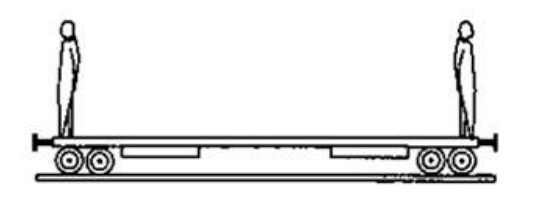# Solve the following :

Question:

Two persons each of mass $m$ are standing at the two extremes of a railroad car of mass $M$ resting on a smooth track. The person on left jumps to the left with the horizontal speed u with respect to the state of car before the jump. Thereafter, the other person jumps to the right, again with the same Two persons each of mass $m$ are standing at the two extremes of a railroad car of mass $M$ resting on a smooth track. The person on left jumps to the left with the horizontal speed u with respect to the state of car before the jump. Thereafter, the other person jumps to the right, again with the sameSolution:

After left person jumps,

$0=m u+(M+m) V_{1}$

$V_{1}=\frac{-m u}{(M+m)}$

$\mathrm{u}$ is left, $V_{1}$ is right

After riaht person iumbs

$0=m u+M V_{2}$

After riqht person jumps

$V_{1}=\frac{-m u}{M}$

$\mathrm{u}$ is left, $V_{2}$ is right

(as $V_{2}>V_{1} V_{\text {Net }}$ is left)

Net velocity $=\frac{m u}{M}-\frac{m u}{M+m}$

$=\frac{m^{2} u}{M(m+M)}$ towards left